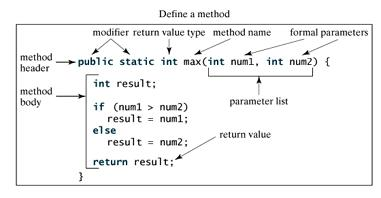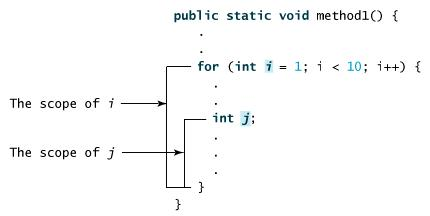Java 方法

• println() 是一个方法
• System 是系统类
• out 是标准输出对象

方法是什么呢？

Java 方法是语句的集合，它们在一起执行一个功能

• 方法是解决一类问题的步骤的有序组合
• 方法包含于类或对象中
• 方法在程序中被创建，在其他地方被引用

1. 使程序变得更简短而清晰
2. 有利于程序维护
3. 可以提高程序开发的效率
4. 提高了代码的重用性

Java 方法的命名规则

1. 必须以字母、下划线(_) 或 美元(＄) 开头
2. 可以包括数字，但不能以它开头

方法的定义

```修饰符 返回值类型 方法名(参数类型 参数名){
...
方法体
...
return 返回值;
}
```

• 修饰符： 修饰符，这是可选的，告诉编译器如何调用该方法。定义了该方法的访问类型
• 返回值类型 ： 方法可能会返回值。returnValueType 是方法返回值的数据类型。有些方法执行所需的操作，但没有返回值。在这种情况下，returnValueType 是关键字 void
• 方法名： 是方法的实际名称。方法名和参数表共同构成方法签名
• 参数类型： 参数像是一个占位符。当方法被调用时，传递值给参数。这个值被称为实参或变量。参数列表是指方法的参数类型、顺序和参数的个数。参数是可选的，方法可以不包含任何参数
• 方法体： 方法体包含具体的语句，定义该方法的功能```public static int age(int birthday){...}
```

```static float interest(float principal, int year){...}
```

范例

```/** 返回两个整型变量数据的较大值 */
public static int max(int num1, int num2) {
int result;
if (num1 > num2)
result = num1;
else
result = num2;

return result;
}
```

调用方法

Java 支持两种调用方法的方式，根据方法是否返回值来选择

```int larger = max(30, 40);
```

```System.out.println("欢迎访问简单编程！");
```

范例

```public class TestMax {
/** 主方法 */
public static void main(String[] args) {
int i = 5;
int j = 2;
int k = max(i, j);
System.out.println( i + " 和 " + j + " 比较，最大值是：" + k);
}

/** 返回两个整数变量较大的值 */
public static int max(int num1, int num2) {
int result;
if (num1 > num2)
result = num1;
else
result = num2;

return result;
}
}
```

```5 和 2 比较，最大值是：5
```

main 方法是被 JVM 调用的，除此之外，main 方法和其它方法没什么区别

main 方法的头部是不变的，如例子所示，带修饰符 public 和 static,返回 void 类型值，方法名字是 main,此外带个一个 String[] 类型参数，String[] 表明参数是字符串数组

void 关键字

```public class TestVoidMethod {
public static void main(String[] args) {
}

public static void printGrade(double score) {
if (score >= 90.0) {
System.out.println('A');
}
else if (score >= 80.0) {
System.out.println('B');
}
else if (score >= 70.0) {
System.out.println('C');
}
else if (score >= 60.0) {
System.out.println('D');
}
else {
System.out.println('F');
}
}
}
```

```C
```

通过值传递参数

```public static void nPrintln(String message, int n) {

for (int i = 0; i < n; i++) {
System.out.println(message);
}
}
```

```public class TestPassByValue {
public static void main(String[] args) {
int num1 = 1;
int num2 = 2;

System.out.println("交换前 num1 的值为：" +
num1 + " ，num2 的值为：" + num2);

// 调用swap方法
swap(num1, num2);
System.out.println("交换后 num1 的值为：" +
num1 + " ，num2 的值为：" + num2);
}
/** 交换两个变量的方法 */
public static void swap(int n1, int n2) {
System.out.println("\t进入 swap 方法");
System.out.println("\t\t交换前 n1 的值为：" + n1
+ "，n2 的值：" + n2);
// 交换 n1 与 n2的值
int temp = n1;
n1 = n2;
n2 = temp;

System.out.println("\t\t交换后 n1 的值为 " + n1
+ "，n2 的值：" + n2);
}
}
```

```交换前 num1 的值为：1 ，num2 的值为：2
进入 swap 方法
交换前 n1 的值为：1，n2 的值：2
交换后 n1 的值为 2，n2 的值：1

```

方法的重载

```public static double max(double num1, double num2) {
if (num1 > num2)
return num1;
else
return num2;
}
```

Java编译器根据方法签名判断哪个方法应该被调用

变量作用域

for循环的初始化部分声明的变量，其作用范围在整个循环命令行参数的使用

```public class CommandLine {
public static void main(String args[]){
for(int i=0; i<args.length; i++){
System.out.println("args[" + i + "]: " +
args[i]);
}
}
}
```

```\$ javac CommandLine.java
\$ java CommandLine this is a command line 200 -100
args: this
args: is
args: a
args: command
args: line
args: 200
args: -100
```

构造方法

```// 一个简单的构造函数
class MyClass {
int x;

// 以下是构造函数
MyClass() {
x = 10;
}
}
```

```public class ConsDemo {
public static void main(String args[]) {
MyClass t1 = new MyClass();
MyClass t2 = new MyClass();
System.out.println(t1.x + " " + t2.x);
}
}
```

```// 一个简单的构造函数
class MyClass {
int x;

// 以下是构造函数
MyClass(int i ) {
x = i;
}
}
```

```public class ConsDemo {
public static void main(String args[]) {
MyClass t1 = new MyClass( 10 );
MyClass t2 = new MyClass( 20 );
System.out.println(t1.x + " " + t2.x);
}
}
```

```10 20
```

可变参数

Java 支持传递同类型的可变参数给一个方法

```typeName... parameterName
```

```public class VarargsDemo {
public static void main(String args[]) {
// 调用可变参数的方法
printMax(34, 3, 3, 2, 56.5);
printMax(new double[]{1, 2, 3});
}

public static void printMax( double... numbers) {
if (numbers.length == 0) {
System.out.println("No argument passed");
return;
}

double result = numbers;

for (int i = 1; i <  numbers.length; i++){
if (numbers[i] >  result) {
result = numbers[i];
}
}
System.out.println("The max value is " + result);
}
}
```

```The max value is 56.5
The max value is 3.0
```

finalize() 方法

Java 允许定义这样的方法，它在对象被垃圾收集器析构(回收)之前调用，这个方法叫做 finalize( )，它用来清除回收对象

语法

```protected void finalize()
{
// 在这里终结代码
}
```

```public class FinalizationDemo {
public static void main(String[] args) {
Cake c1 = new Cake(1);
Cake c2 = new Cake(2);
Cake c3 = new Cake(3);

c2 = c3 = null;
System.gc(); //调用Java垃圾收集器
}
}

class Cake extends Object {
private int id;
public Cake(int id) {
this.id = id;
System.out.println("Cake Object " + id + "is created");
}

protected void finalize() throws java.lang.Throwable {
super.finalize();
System.out.println("Cake Object " + id + "is disposed");
}
}
```

```\$ javac FinalizationDemo.java
\$ java FinalizationDemo
Cake Object 1is created
Cake Object 2is created
Cake Object 3is created
Cake Object 3is disposed
Cake Object 2is disposed
```

Java 基础教程

简单教程，简单编程 - IT 入门首选站